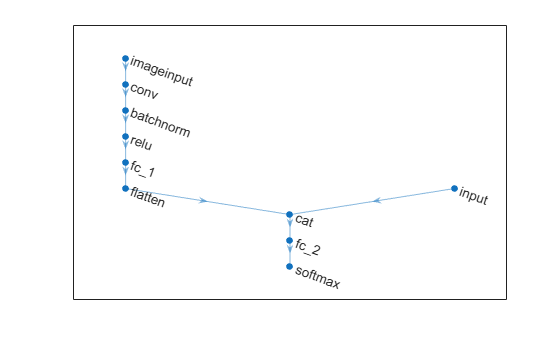## Syntax

``netUpdated = addInputLayer(net,layer)``
``netUpdated = addInputLayer(net,layer,inputName)``
``netUpdated = addInputLayer(___,Initialize=tf)``

## Description

example

````netUpdated = addInputLayer(net,layer)` adds the input layer `layer` to the network `net` by connecting the input layer to the first unconnected input in `net`. If the updated network supports automatic initialization, then the function automatically initializes the learnable parameters of the network.```

example

````netUpdated = addInputLayer(net,layer,inputName)` also specifies which unconnected input the function connects to the input layer.```
````netUpdated = addInputLayer(___,Initialize=tf)` also specifies whether to initialize the output network using any of the previous syntaxes.```

## Examples

collapse all

Create an uninitialized `dlnetwork` object that does not have an input layer.

```layers = [ convolution2dLayer(5,20) reluLayer maxPooling2dLayer(2,Stride=2) fullyConnectedLayer(10) softmaxLayer]; net = dlnetwork(layers,Initialize=false)```
```net = dlnetwork with properties: Layers: [5×1 nnet.cnn.layer.Layer] Connections: [4×2 table] Learnables: [4×3 table] State: [0×3 table] InputNames: {'conv'} OutputNames: {'softmax'} Initialized: 0 View summary with summary. ```

Create an image input layer with an input size of `[28 28 1]`.

`layer = imageInputLayer([28 28 1],Normalization="none");`

Add the input layer to the network.

`net = addInputLayer(net,layer)`
```net = dlnetwork with properties: Layers: [6×1 nnet.cnn.layer.Layer] Connections: [5×2 table] Learnables: [4×3 table] State: [0×3 table] InputNames: {'imageinput'} OutputNames: {'softmax'} Initialized: 1 View summary with summary. ```

View the `Initialized` property. Because the network contains all the information required for initialization, the output network is initialized.

`net.Initialized`
```ans = logical 1 ```

Create an uninitialized `dlnetwork` object that has two unconnected inputs.

```layers = [ convolution2dLayer(5,16,Name="conv") batchNormalizationLayer reluLayer fullyConnectedLayer(50) flattenLayer concatenationLayer(1,2,Name="cat") fullyConnectedLayer(10) softmaxLayer]; net = dlnetwork(layers,Initialize=false);```

Create an image input layer to connect to the convolution layer.

`layer = imageInputLayer([28 28 1],Normalization="none");`

Connect the image input layer to the `"conv"` layer. Because the network does not contain the information required to initialize the network, the returned network is uninitialized.

`net = addInputLayer(net,layer,"conv")`
```net = dlnetwork with properties: Layers: [9×1 nnet.cnn.layer.Layer] Connections: [8×2 table] Learnables: [8×3 table] State: [2×3 table] InputNames: {'imageinput' 'cat/in2'} OutputNames: {'softmax'} Initialized: 0 View summary with summary. ```

Create a feature input layer to connect to the second input of the concatenation layer.

`layer = featureInputLayer(1);`

Connect the feature input layer to the `"in2"` input of the `"cat"` layer. Because the network now contains the information required to initialize the network, the returned network is initialized.

`net = addInputLayer(net,layer,"cat/in2")`
```net = dlnetwork with properties: Layers: [10×1 nnet.cnn.layer.Layer] Connections: [9×2 table] Learnables: [8×3 table] State: [2×3 table] InputNames: {'imageinput' 'input'} OutputNames: {'softmax'} Initialized: 1 View summary with summary. ```

Visualize the network in a plot.

```figure plot(net)```## Input Arguments

collapse all

Neural network, specified as a `dlnetwork` object.

Input layer to add, specified as a `Layer` object.

Unconnected network input which the function connects the layer, specified as a string scalar, a character vector, or a cell array containing a character vector.

Data Types: `char` | `string` | `cell`

Flag to initialize the learnable parameters of the output network, specified as one of these values:

• `"auto"` — If the network does contains all the required information for initialization, then the function initializes the output network. Otherwise, the function does not initialize the output network..

• `1` (`true`) — Initialize the output network. If the network does not contain all the required information for initialization, then the function errors.

• `0` (`false`) — Do not initialize the output network.

## Output Arguments

collapse all

Updated network, returned as a `dlnetwork` object.

The function reorders the layers in the `Layers` property of the network such that the input layer appears immediately before the layer that it is connected to.

## Version History

Introduced in R2022b